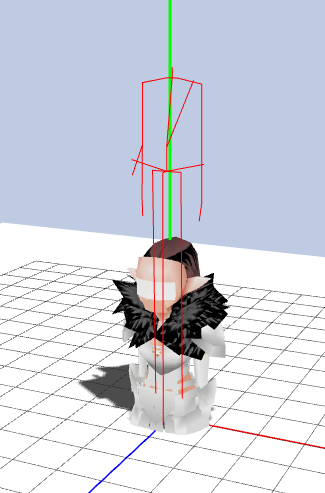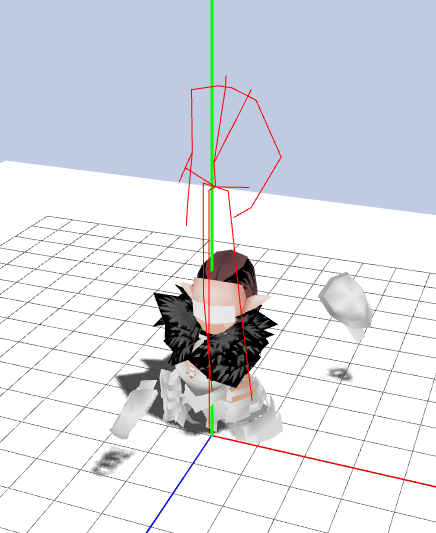1. 播放骨骼动画时，角色模型被肢解了。
2. 我希望通过手动拖拽进度条来控制动画，但是我不知道该怎么实现。
3. 模型的影子会突然消失。
4. 角色模型的部件之间有裂缝。
5. 人类/精灵的模型原点在肚脐处，不在脚底。这导致骨骼动画绑定异常。

## 项目介绍

“洛奇(Mabinogi)”是一款韩国NEXON公司出品的生活类3DMMORPG，我从大学开始玩这个游戏，至今断断续续也有好些年了。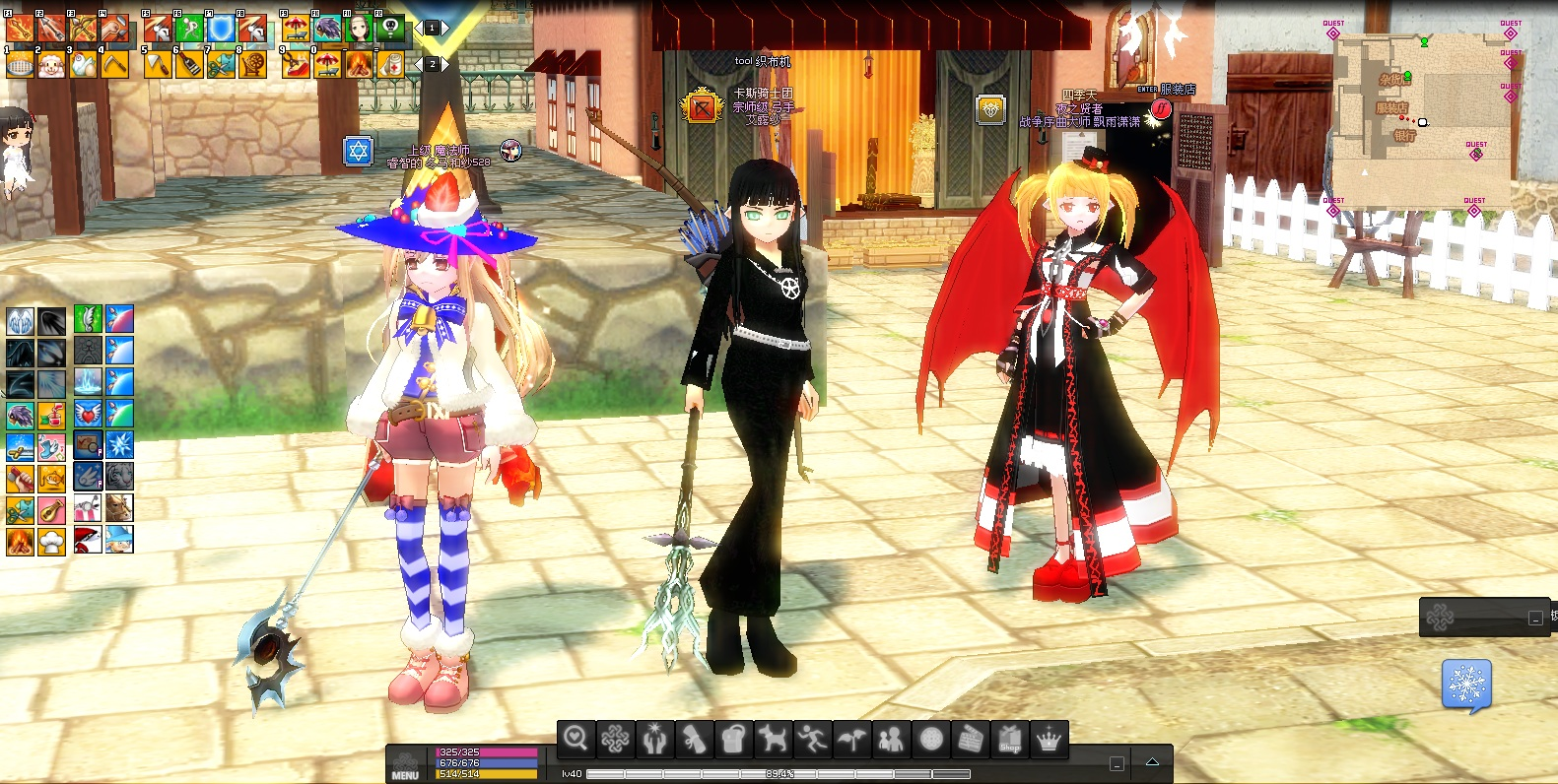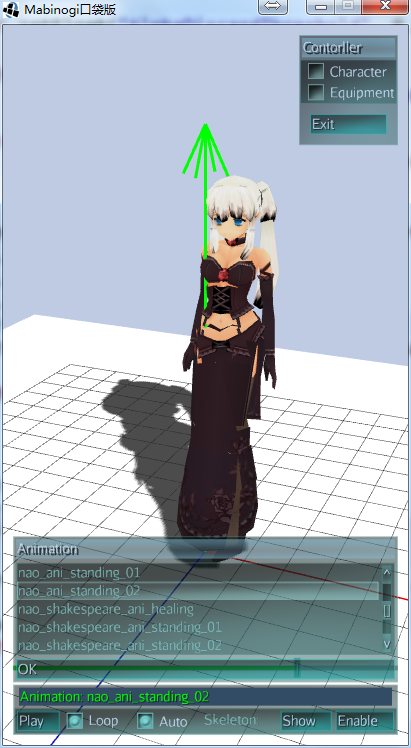## 技术难点

### Pack文件解析

Mabinogi的大部分游戏资源是存储在.pack包中的，其中的文件数据经过了加密、压缩。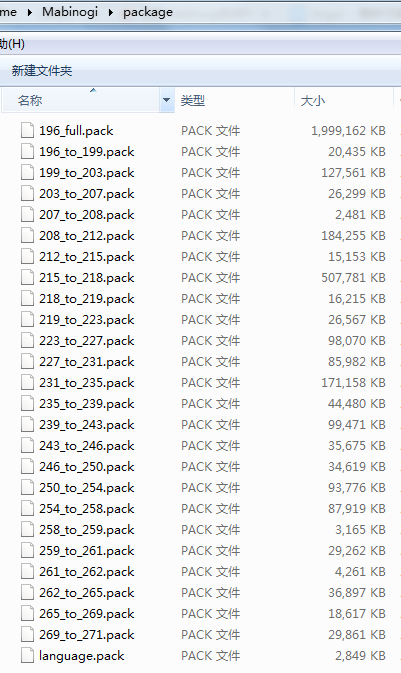Pack文件的解析过程并不复杂，难点在于提取出Pack中的文件数据。Pack中的文件首先被zlib算法压缩，然后又使用mt19937伪随机算法进行XOR加密。

https://github.com/darknesstm/MabinogiPackageTool

net.jmecn.mabi.utils.MersenneTwister.java

``````long seed = ((long) entry.Seed << 7) ^ 0xA9C36DE1L;
MersenneTwister mt = new MersenneTwister(seed);
for (int i = 0; i < data.length; i++) {
data[i] = (byte) (data[i] ^ mt.genrandInt32());
}
``````

Pack包的解析类为：

net.jmecn.mabi.pack.PackFile.java

``````String pack = "/home/yan/Apps/Mabinogi/package/language.pack";
assetManager.registerLocator(pack, PackLocator.class);
``````

### 3D模型文件

Mabinogi的3D模型分别保存为3种格式：pmg、frm、ani。

frm文件

frm文件中保存了模型的骨骼信息，定义了由最大128根骨头组成的层次关系。每个骨头都有保留有自己的命名、位置等信息。

pmg文件

pmg文件中保存了模型的网格、材质等信息。一个pmg文件中可能包含多个网格，每个网格对应一个材质。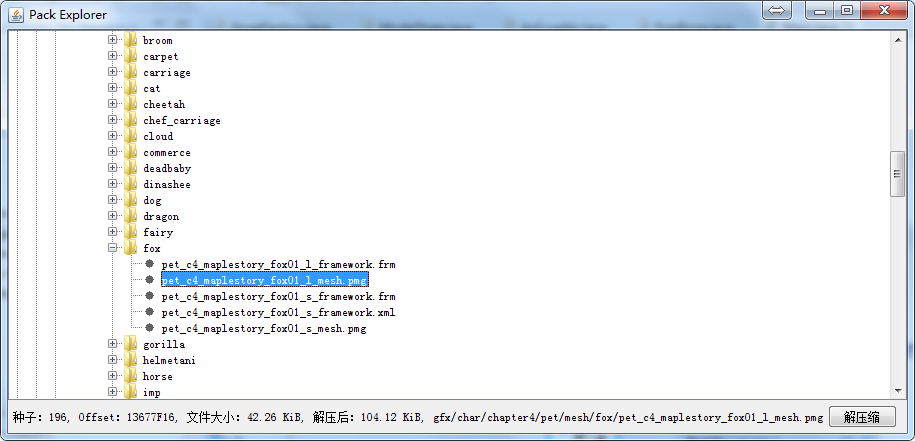ani文件

ani文件中保存了模型的骨骼动画数据，每个ani文件只保存一个动画。如果一个模型有站立、坐下、攻击等多个动画，就会保存为多个ani文件。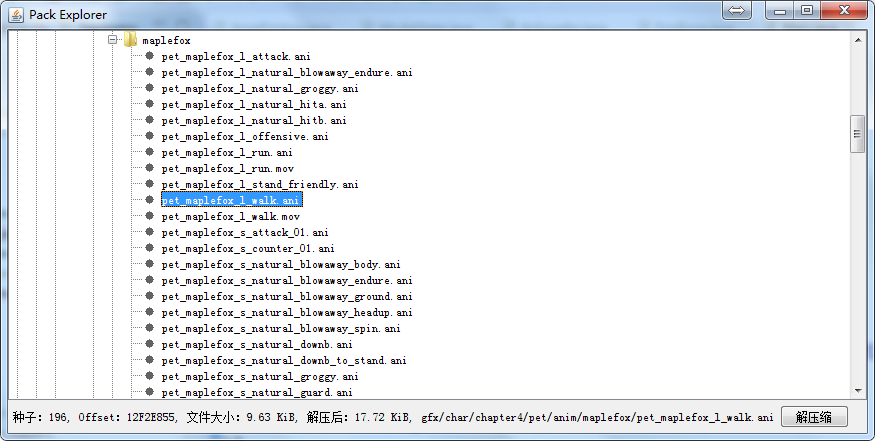``````// Mabinogi plugin
``````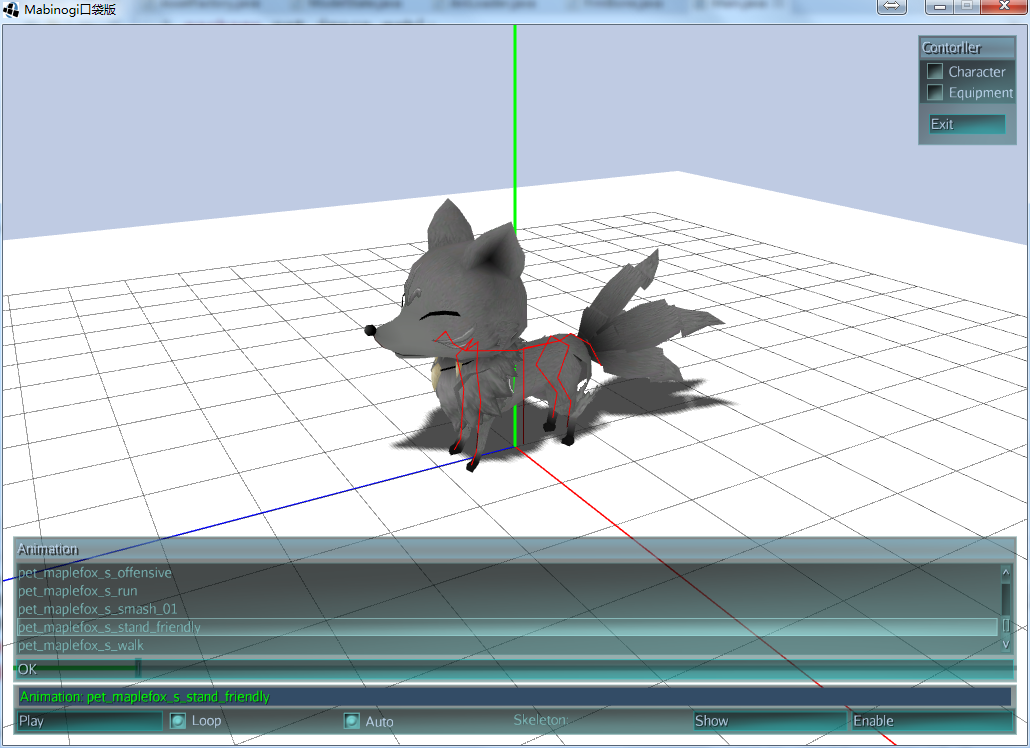### 线性变换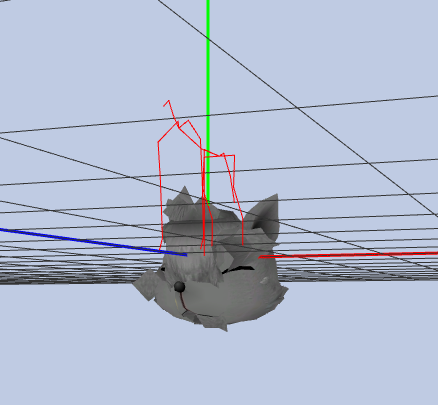``````Geometry geom = new Geometry(pmg.meshName, mesh);
geom.setLocalRotation(pmg.majorMatrix.toRotationQuat());
geom.setLocalTranslation(pmg.majorMatrix.toTranslationVector());
geom.setLocalScale(pmg.majorMatrix.toScaleVector());
``````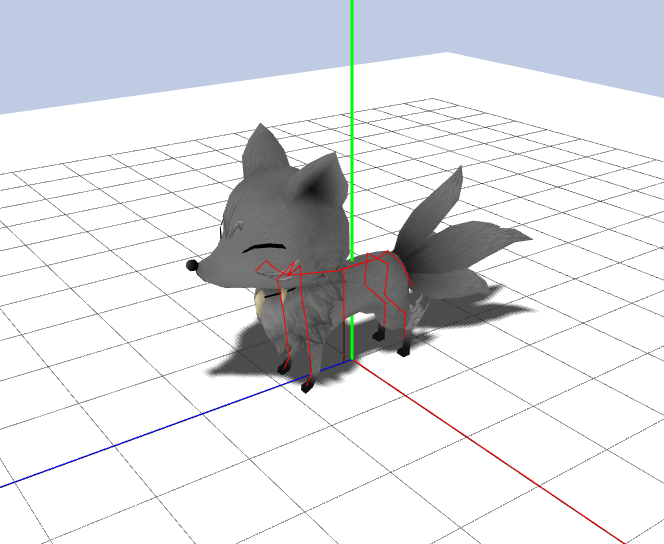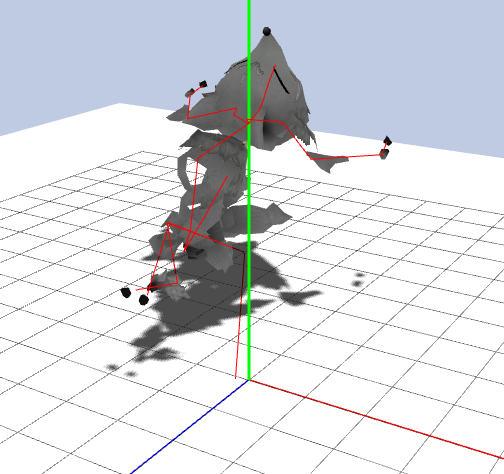### 动画数据

``````/**
*
* Modify the bone which this track modifies in the skeleton to contain
* the correct animation transforms for a given time.
* The transforms can be interpolated in some method from the keyframes.
*
* @param time the current time of the animation
* @param weight the weight of the animation
* @param control
* @param channel
* @param vars
*/
public void setTime(float time, float weight, AnimControl control, AnimChannel channel, TempVars vars) {
BitSet affectedBones = channel.getAffectedBones();
if (affectedBones != null && !affectedBones.get(targetBoneIndex)) {
return;
}

Bone target = control.getSkeleton().getBone(targetBoneIndex);

Vector3f tempV = vars.vect1;
Vector3f tempS = vars.vect2;
Quaternion tempQ = vars.quat1;
Vector3f tempV2 = vars.vect3;
Vector3f tempS2 = vars.vect4;
Quaternion tempQ2 = vars.quat2;

int lastFrame = times.length - 1;
if (time < 0 || lastFrame == 0) {
rotations.get(0, tempQ);
translations.get(0, tempV);
if (scales != null) {
scales.get(0, tempS);
}
} else if (time >= times[lastFrame]) {
rotations.get(lastFrame, tempQ);
translations.get(lastFrame, tempV);
if (scales != null) {
scales.get(lastFrame, tempS);
}
} else {
int startFrame = 0;
int endFrame = 1;
// use lastFrame so we never overflow the array
int i;
for (i = 0; i < lastFrame && times[i] < time; i++) {
startFrame = i;
endFrame = i + 1;
}

float blend = (time - times[startFrame])
/ (times[endFrame] - times[startFrame]);

rotations.get(startFrame, tempQ);
translations.get(startFrame, tempV);
if (scales != null) {
scales.get(startFrame, tempS);
}
rotations.get(endFrame, tempQ2);
translations.get(endFrame, tempV2);
if (scales != null) {
scales.get(endFrame, tempS2);
}
tempQ.nlerp(tempQ2, blend);
tempV.interpolateLocal(tempV2, blend);
tempS.interpolateLocal(tempS2, blend);
}

target.blendAnimTransforms(tempV, tempQ, scales != null ? tempS : null, weight);

}
``````

Bone中根据BoneTrack计算的数据来改变自身姿态的代码如下：

``````/**
* Blends the given animation transform onto the bone's local transform.
* <p>
* Subsequent calls of this method stack up, with the final transformation
* of the bone computed at {@link #updateModelTransforms() } which resets
* the stack.
* <p>
* E.g. a single transform blend with weight = 0.5 followed by an
* updateModelTransforms() call will result in final transform = transform * 0.5.
* Two transform blends with weight = 0.5 each will result in the two
* transforms blended together (nlerp) with blend = 0.5.
*
* @param translation The translation to blend in
* @param rotation The rotation to blend in
* @param scale The scale to blend in
* @param weight The weight of the transform to apply. Set to 1.0 to prevent
* any other transform from being applied until updateModelTransforms().
*/
void blendAnimTransforms(Vector3f translation, Quaternion rotation, Vector3f scale, float weight) {
if (userControl) {
return;
}

if (weight == 0) {
// Do not apply this transform at all.
return;
}

if (currentWeightSum == 1){
return; // More than 2 transforms are being blended
} else if (currentWeightSum == -1 || currentWeightSum == 0) {
// Set the transform fully
localRot.set(bindRot).multLocal(rotation);
if (scale != null) {
localScale.set(bindScale).multLocal(scale);
}
// Set the weight. It will be applied in updateModelTransforms().
currentWeightSum = weight;
} else {
// The weight is already set.
// Blend in the new transform.
TempVars vars = TempVars.get();

Vector3f tmpV = vars.vect1;
Vector3f tmpV2 = vars.vect2;
Quaternion tmpQ = vars.quat1;

localPos.interpolateLocal(tmpV, weight);

tmpQ.set(bindRot).multLocal(rotation);
localRot.nlerp(tmpQ, weight);

if (scale != null) {
tmpV2.set(bindScale).multLocal(scale);
localScale.interpolateLocal(tmpV2, weight);
}

// Ensures no new weights will be blended in the future.
currentWeightSum = 1;

vars.release();
}
}
``````

BoneTrack中`setTime`方法的作用，就是根据动画播放的时间`time`，查找具体的位移数据`V[t]`。然后Bone的`blendAnimTransforms`方法的作用，就是根据Bone的初始位移`Va`和BoneTrack提供的位移数据`V[t]`，计算出实际的位移`Va + v[t]`

``````        tmpV.set(bindPos).addLocal(translation);
localPos.interpolateLocal(tmpV, weight);
``````

``````        tmpQ.set(bindRot).multLocal(rotation);
localRot.nlerp(tmpQ, weight);

if (scale != null) {
tmpV2.set(bindScale).multLocal(scale);
localScale.interpolateLocal(tmpV2, weight);
}
``````

jME3中骨骼动画的计算方式我差不多明白了，下面再来看看ani文件中的数据是否有问题。

``````	/**
* 根据骨骼姿态，修正动画数据。
*/
Vector3f bindPosition = new Vector3f();
Quaternion bindRotationI = new Quaternion();// 逆旋转
Quaternion tmpQ = new Quaternion();

for (int i = 0; i < aniFile.boneCount; i++) {
AniTrack aniTrack = aniFile.aniTracks[i];

// ...

// 骨骼的初始动作
if (skeleton != null) {
Bone bone = skeleton.getBone(i);
bindPosition.set(bone.getBindPosition());
bindRotationI.set(bone.getBindRotation().inverse());// 计算逆旋转
}

BoneTrack track = new BoneTrack(i);

float[] times = new float[aniTrack.frameCount];
Vector3f[] translations = new Vector3f[aniTrack.frameCount];
Quaternion[] rotations = new Quaternion[aniTrack.frameCount];
Vector3f[] scales = new Vector3f[aniTrack.frameCount];

for (int j = 0; j < aniTrack.frameCount; j++) {
AniFrame aniFrame = aniTrack.aniFrames[j];
times[j] = (float) aniFrame.frameNo / aniFile.framePerSecond;
translations[j] = new Vector3f(aniFrame.x, aniFrame.y, aniFrame.z);
rotations[j] = new Quaternion(aniFrame.qx, aniFrame.qy, aniFrame.qz, -aniFrame.qw);
scales[j] = new Vector3f(1f, 1f, 1f);

if (skeleton != null) {
// 还原位移
translations[j].subtractLocal(bindPosition);

// 还原旋转
bindRotationI.mult(rotations[j], tmpQ);
rotations[j].set(tmpQ);
}
}

track.setKeyframes(times, translations, rotations, scales);
}
``````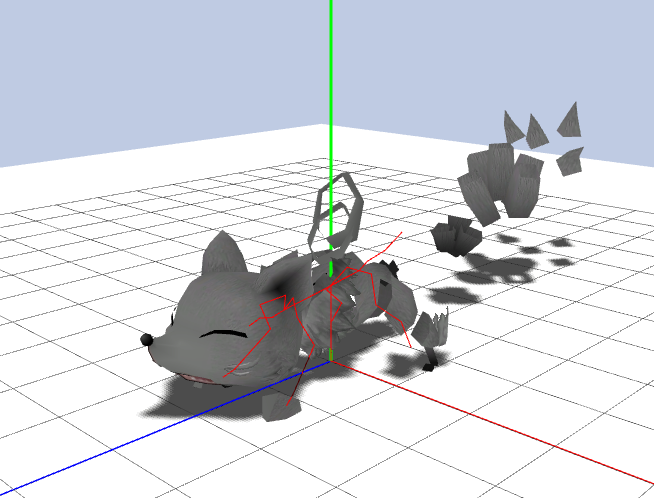### 骨骼蒙皮

`````` /**
* Stores the skinning transform in the specified Matrix4f.
* The skinning transform applies the animation of the bone to a vertex.
*
* This assumes that the world transforms for the entire bone hierarchy
* have already been computed, otherwise this method will return undefined
* results.
*
* @param outTransform
*/
void getOffsetTransform(Matrix4f outTransform, Quaternion tmp1, Vector3f tmp2, Vector3f tmp3, Matrix3f tmp4) {
// Computing scale
Vector3f scale = modelScale.mult(modelBindInverseScale, tmp3);

// Computing rotation
Quaternion rotate = modelRot.mult(modelBindInverseRot, tmp1);

// Computing translation
// Translation depend on rotation and scale
Vector3f translate = modelPos.add(rotate.mult(scale.mult(modelBindInversePos, tmp2), tmp2), tmp2);

// Populating the matrix
outTransform.setTransform(translate, scale, rotate.toRotationMatrix(tmp4));
}
``````

``````Geometry geom = new Geometry(pmg.meshName, mesh);
/**
* 该空间变化应该应用到网格顶点上，否则骨骼蒙皮动画无法正常工作。
*/
//geom.setLocalRotation(pmg.majorMatrix.toRotationQuat());
//geom.setLocalTranslation(pmg.majorMatrix.toTranslationVector());
//geom.setLocalScale(pmg.majorMatrix.toScaleVector());
``````

``````/**
* 创建网格
*
* @param pmg
* @return
*/
public static Mesh buildMesh(PmGeometry pmg) {
Mesh mesh = new Mesh();
// ...
Matrix4f transform = pmg.majorMatrix;
Matrix3f rotation = transform.toRotationMatrix();
for (int i = 0; i < pmg.vertexCount; i++) {

/**
* 为了保证骨骼蒙皮动画正常，顶点和法线要通过pmg.majorMatrix进行空间转换。
*/
vertexes[i] = transform.mult(pmg.verts[i].getPosition());
normals[i] = rotation.mult(pmg.verts[i].getNormal());

//vertexes[i] = pmg.verts[i].getPosition();
//normals[i] = pmg.verts[i].getNormal();
vertexColors[i] = pmg.verts[i].getColor();
texCoords[i] = pmg.verts[i].getTexCoord();
}
//...
return mesh;
}
``````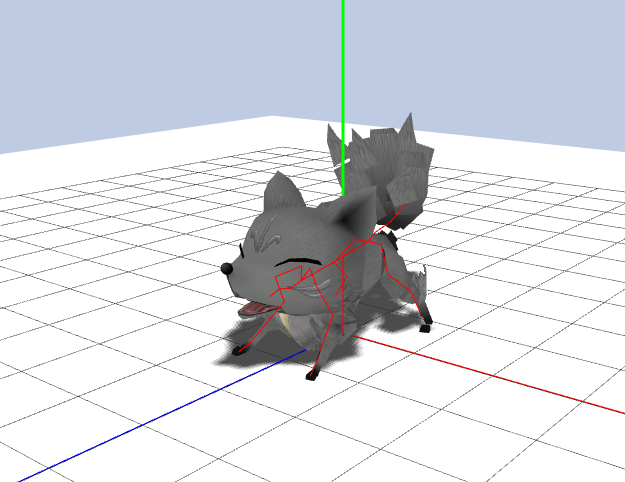### 精灵和人类的原点不对